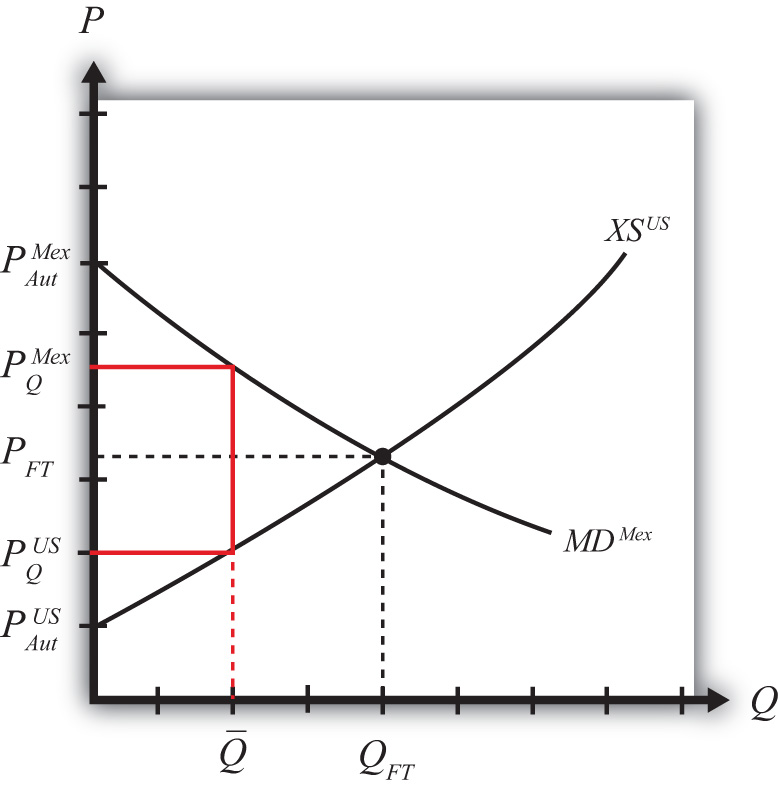This is “Import Quotas: Large Country Price Effects”, section 7.10 from the book Policy and Theory of International Trade (v. 1.0). For details on it (including licensing), click here.

Has this book helped you? Consider passing it on:
Creative Commons supports free culture from music to education. Their licenses helped make this book available to you.
DonorsChoose.org helps people like you help teachers fund their classroom projects, from art supplies to books to calculators.

## 7.10 Import Quotas: Large Country Price Effects

### Learning Objectives

1. Identify the effects of an import quota on prices in both countries and the quantity traded.
2. Know the equilibrium conditions that must prevail in a quota equilibrium.

Suppose Mexico, the importing country in free trade, imposes a binding import quota on wheat. The quota will restrict the flow of wheat across the border. As a result, the supply of wheat to the Mexican market will fall, and if the price remains the same, it will cause excess demand for wheat in the market. The excess demand will induce an increase in the price of wheat. Since wheat is homogeneous and the market is perfectly competitive, the price of all wheat sold in Mexico, both Mexican wheat and U.S. imports, will rise in price. The higher price will, in turn, reduce demand and increase domestic supply, causing a reduction in Mexico’s import demand.

The restricted wheat supply to Mexico will shift supply back to the U.S. market. Since Mexico is assumed to be a large importer, the supply shifted back to the U.S. market will generate excess supply in the U.S. market at the original price and cause a reduction in the U.S. price. The lower price will, in turn, reduce U.S. supply, raise U.S. demand, and cause a reduction in U.S. export supply.

These price effects are identical in direction to the price effects of an import tax, a voluntary export restraint, and an export tax.

A new quota equilibrium will be reached when the following two conditions are satisfied:

$MDMex(PQMex)=Q¯$

and

$XSUS(PQUS)=Q¯,$

where $Q¯$ is the quantity at which the quota is set,$PQMex$ is the price in Mexico after the quota, and $PQUS$ is the price in the United States after the quota.

The first condition says that the price must change in Mexico such that import demand falls to the quota level $Q¯$. In order for this to occur, the price in Mexico rises. The second condition says that the price must change in the United States such that export supply falls to the quota level $Q¯$. In order for this to occur, the price in the United States falls.

The quota equilibrium is depicted on the graph in Figure 7.24 "Depicting a Quota Equilibrium: Large Country Case". The Mexican price of wheat rises from PFT to $PQM$, which is sufficient to reduce its import demand from QFT to $Q¯$. The U.S. price of wheat falls from PFT to $PQUS$, which is sufficient to reduce its export supply from QFT to $Q¯$.

Figure 7.24 Depicting a Quota Equilibrium: Large Country CaseNotice that there is a unique set of prices that satisfies the equilibrium conditions for every potential quota that is set. If the quota were set lower than $Q¯$, the price wedge would rise, causing a further increase in the Mexican price and a further decrease in the U.S. price.

At the extreme, if the quota were set equal to zero, then the prices in each country would revert to their autarky levels. In this case, the quota would prohibit trade.

### Key Takeaways

• An import quota will raise the domestic price and, in the case of a large country, lower the foreign price.
• The difference between the foreign and domestic prices after the quota is implemented is known as a quota rent.
• An import quota will reduce the quantity of imports to the quota amount.

### Exercise

1. Jeopardy Questions. As in the popular television game show, you are given an answer to a question and you must respond with the question. For example, if the answer is “a tax on imports,” then the correct question is “What is a tariff?”

1. The direction of change of domestic producer surplus when an import quota is implemented by a domestic country.
2. The direction of change of the domestic price after a binding import quota is implemented by a domestic country.
3. The direction of change of the foreign price after a binding import quota is implemented by a large domestic country.
4. Of increase, decrease, or stay the same, this is the effect on the domestic price after a nonbinding import quota is implemented by a domestic country.
5. The term used to describe a zero quota that eliminates trade.
6. Of increase, decrease, or stay the same, this is the effect on the price of U.S.-made automobiles if the United States restricts the quantity of imported foreign automobiles.
7. Of increase, decrease, or stay the same, this is the effect on the quantity of wheat imports if a binding import quota is implemented.
8. Of increase, decrease, or stay the same, this is the effect on foreign exports of wheat if a binding import quota is implemented by an importing country.